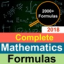Hlavní navigace

#ALL Maths Formulas Guide 1.2

All Maths Formulas professional Guide is simple yet amazing application for students, scientist, engineers as well as for analysts. Essential mathematics formulas a complete lists all the formula related to Integrals, Polynomials, Analytic Geometry, Calculus, Algebra, Probability, Statistics, Trigonometry, Matrices, Equations, Integration, Derivative, Limits, Geometry, and Logic. Almost cover all mathematical formulas.
Essential Mathematics Formulas Cheat Sheet of 2000+ maths formulas.

All Maths Formulas professional Guide Application includes:

* Basics
* Numbers
* Algebra
* Derivatives Definition and Notation
* Interpretation of the Derivative
* Basic Properties and Formulas
* Common Derivatives
* Chain Rule Variants
* Integrals Definitions
* Higher Order Derivatives
* Implicit Differentiation
* Increasing/Decreasing – Concave Up/Concave Down
* Fundamental Theorem of Calculus
* Extrema
* Mean Value Theorem
* Newton’s Method
* Related Rates
* Trigonometry Functions
* Special Angles
* Trigonometric Function Values in Quadrants II, III, and IV
* The Unit Circle
* Double Angle Formulas
* Half Angle Formulas
* Power Reducing Formulas
* Product‐to‐Sum Formulas
* Co-functions Formulas
* Law of Sines
* Law of Cosines
* Law of Tangents
* Pythagorean Identities (for any angle θ)
* Mollweide’s Formula
* Optimization
* Factoring Formulas
* Square Root Property
* Absolute Value Equations/Inequalities
* Completing the Square
* Functions and Graphs
* Integrals Definitions
* Common Integrals
* Trigonometry
* Calculus
* Sets
* Matrix
* Geometry
* Statistics
* Probability
* Vectors
* Series
* Boolean Algebra
* Analytical Geometry
* Integration
* Partial Derivatives
* Series & Sequences

And much more

## Celkové hodnocení

Průměr hodnocení
3

Pro hodnocení programu se prosím nejprve

## Souhrnné informace o ALL Maths Formulas Guide

1.2

ano

44,66 MB

• Angličtina
• #### Staženo

0× celkem
0× tento měsíc

20. 10. 2019

## Něco jsme propásli?

Dejte nám vědět. Upozornit redakci Stahuj
Velice děkujeme za Vaše podněty